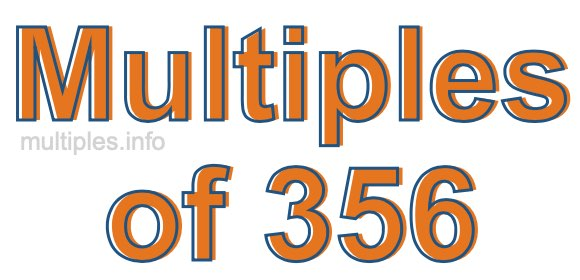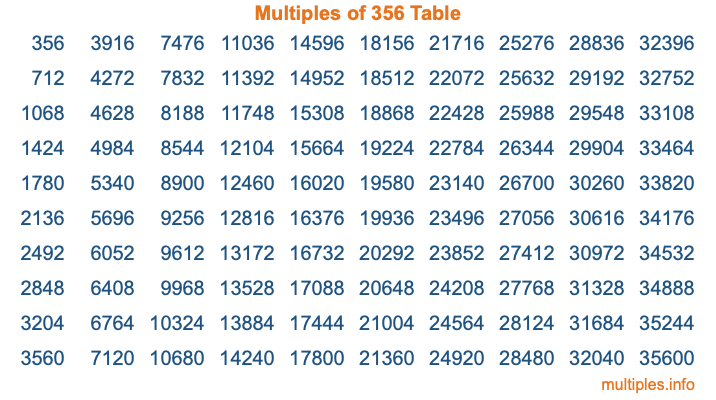Multiples of 356Welcome to the Multiples of 356 page. Here we will first teach you everything you will ever need to know about the multiples of 356, and then give you a study guide summary of everything we taught you to make sure you remember it all. Use this page to look up facts and learn information about the multiples of 356. This page will make you a multiples of three hundred fifty-six expert!

Definition of Multiples of 356
Multiples of 356 are all the numbers that when divided by 356 equal an integer. Each of the multiples of 356 are called a multiple. A multiple of 356 is created by multiplying 356 by an integer.

Therefore, to create a list of multiples of 356, you start with 1 multiplied by 356, then 2 multiplied by 356, then 3 multiplied by 356, and so on for as long as you want. Thus, the list of the first five multiples of 356 is 356, 712, 1068, 1424, and 1780. To see a larger list of multiples of 356, see the printable image of Multiples of 356 further down on this page. We also have a category where you can choose any nth multiple of 356.

Multiples of 356 Checker
The Multiples of 356 Checker below checks to see if any number of your choice is a multiple of 356. In other words, it checks to see if there is any number (integer) that when multiplied by 356 will equal your number. To do that, we divide your number by 356. If the the quotient is an integer, then your number is a multiple of 356.

Is  a multiple of 356?

Least Common Multiple of 356 and ...
A Least Common Multiple (LCM) is the lowest multiple that two or more numbers have in common. This is also called the smallest common multiple or lowest common multiple and is useful to know when you are adding our subtracting fractions. Enter one or more numbers below (356 is already entered) to find the LCM.

Check out our LCM Calculator if you need more details about the Least Common Multiple or if you need the LCM for different numbers for adding and subtraction fractions.

nth Multiple of 356
As we stated above, 356 is the first multiple of 356, 712 is the second multiple of 356, 1068 is the third multiple of 356, and so on. Enter a number below to find the nth multiple of 356.

th multiple of 356

Multiples of 356 vs Factors of 356
356 is a multiple of 356 and a factor of 356, but that is where the similarities end. All postive multiples of 356 are 356 or greater than 356. All positive factors of 356 are 356 or less than 356.

Below is the beginning list of multiples of 356 and the factors of 356 so you can compare:

Multiples of 356: 356, 712, 1068, 1424, 1780, etc.

Factors of 356: 1, 2, 4, 89, 178, 356

As you can see, the multiples of 356 are all the numbers that you can divide by 356 to get a whole number. The factors of 356, on the other hand, are all the whole numbers that you can multiply by another whole number to get 356.

It's also interesting to note that if a number (x) is a factor of 356, then 356 will also be a multiple of that number (x).

Multiples of 356 vs Divisors of 356
The divisors of 356 are all the integers that 356 can be divided by evenly. Below is a list of the divisors of 356.

Divisors of 356: 1, 2, 4, 89, 178, 356

The interesting thing to note here is that if you take any multiple of 356 and divide it by a divisor of 356, you will see that the quotient is an integer.

Multiples of 356 Table
Below is an image of the first 100 multiples of 356 in a table. The table is in chronological order, column by column. The first column has the first ten multiples of 356, the second column has the next ten multiples of 356, and so on.The Multiples of 356 Table is also referred to as the 356 Times Table or Times Table of 356. You are welcome to print out our table for your studies.

Negative Multiples of 356
Although not often discussed or needed in math, it is worth mentioning that you can make a list of negative multiples of 356 by multiplying 356 by -1, then by -2, then by -3, and so on, to get the following list of negative multiples of 356:

-356, -712, -1068, -1424, -1780, etc.

Multiples of 356 Summary
Below is a summary of important Multiples of 356 facts that we have discussed on this page. To retain the knowledge on this page, we recommend that you read through the summary and explain to yourself or a study partner why they hold true.

There are an infinite number of multiples of 356.

A multiple of 356 divided by 356 will equal a whole number.

356 divided by a factor of 356 equals a divisor of 356.

The nth multiple of 356 is n times 356.

The largest factor of 356 is equal to the first positive multiple of 356.

356 is a multiple of every factor of 356.

356 is a multiple of 356.

A multiple of 356 divided by a divisor of 356 equals an integer.

356 divided by a divisor of 356 equals a factor of 356.

Any integer times 356 will equal a multiple of 356.

Multiples of a Number
Here you can get the multiples of another number, all with the same attention to detail as we did for multiples of 356 on this page.

Multiples of
Multiples of 357
Did you find our page about multiples of three hundred fifty-six educational? Do you want more knowledge? Check out the multiples of the next number on our list!